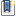File : Details

# Photo-Voltaic (PV) module

Modeling a photovoltaic (solar) battery unitin the Xcos extension of the Scilab application package.
Details
Version
1.0
Author
Zurab Dzhendubaev
Maintainer
Zurab Dzhendubaev
Categories
Supported Scilab Version
6.0
Creation Date
August 8, 2021Description
```            Formulas and parameters were used in the modeling:

VT=VT_ref*(Tcell_K/Tref_K);
I0_T=I0_ref*((Tcell_K/Tref_K)^3)*exp((EgRef/(k1*Tref_K))-(E_g/(k1*Tcell_K)))
Rsh=Rsh_ref*Sref/S

Diode characteristic
Id=I0*[exp(Vd/VT) -1]
where: Id = diode current (A) Vd = diode voltage (V) I0 = diode saturation
current (A)

VT = temperature voltage = k*Tcell_K/q*nI*Ncell*Nser

Tcell_K = cell temperature (K),

k = Boltzman constant = 1.3806e-23 J.K^-1

q = electron charge = 1.6022e-19 C

nI = diode ideality factor

Ncell= number of series-connected cells per module

Nser = number of series-connected modules per string

ki=0.0032
q=1.6e-19
K=1.38e-23
n=1.3
Eg0=1.1
Rs=0.221
Rsh=415.405
Tn=298

Voc=32.9
Isc=8.21
Ns=54            ```Files (5)Screenshot
```
```Screenshot
```
```Screenshot
```
```Source code archive
```
```Screenshot
```
```News (0)Comments (0)Leave a comment
Login withEmail notifications